Скачать презентацию Applications of Equations Lesson 1 2 6

• Количество слайдов: 20Applications of Equations Lesson 1. 2. 6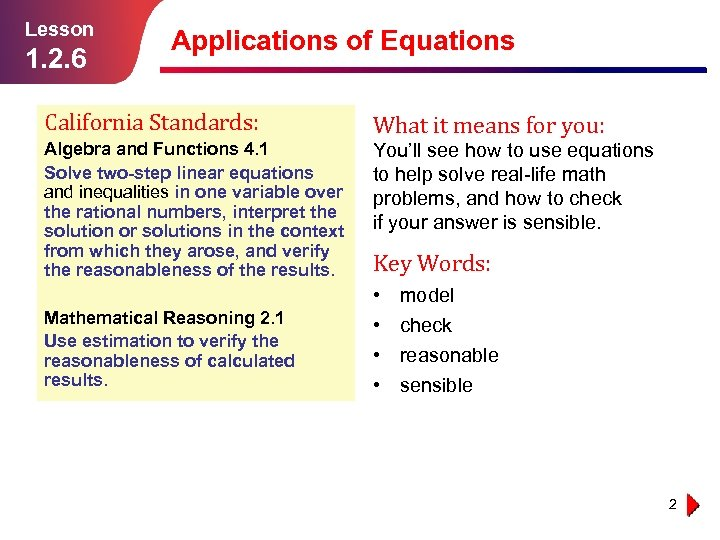Lesson 1. 2. 6 Applications of Equations California Standards: What it means for you: Algebra and Functions 4. 1 Solve two-step linear equations and inequalities in one variable over the rational numbers, interpret the solution or solutions in the context from which they arose, and verify the reasonableness of the results. You’ll see how to use equations to help solve real-life math problems, and how to check if your answer is sensible. Mathematical Reasoning 2. 1 Use estimation to verify the reasonableness of calculated results. Key Words: • • model check reasonable sensible 2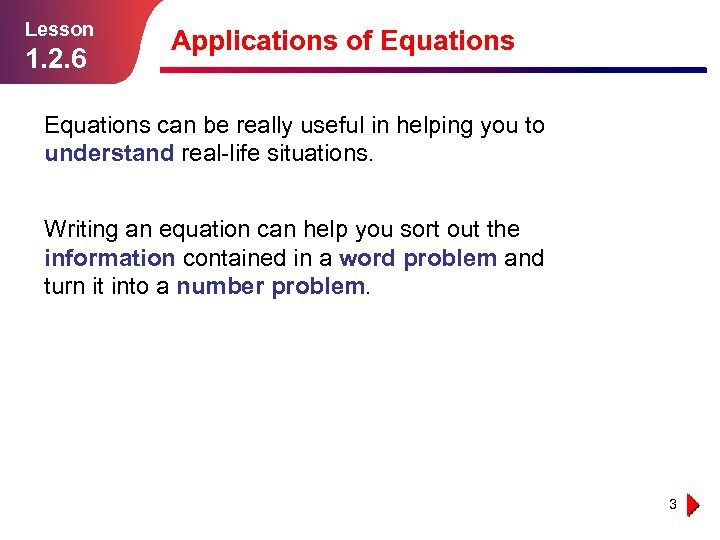Lesson 1. 2. 6 Applications of Equations can be really useful in helping you to understand real-life situations. Writing an equation can help you sort out the information contained in a word problem and turn it into a number problem. 3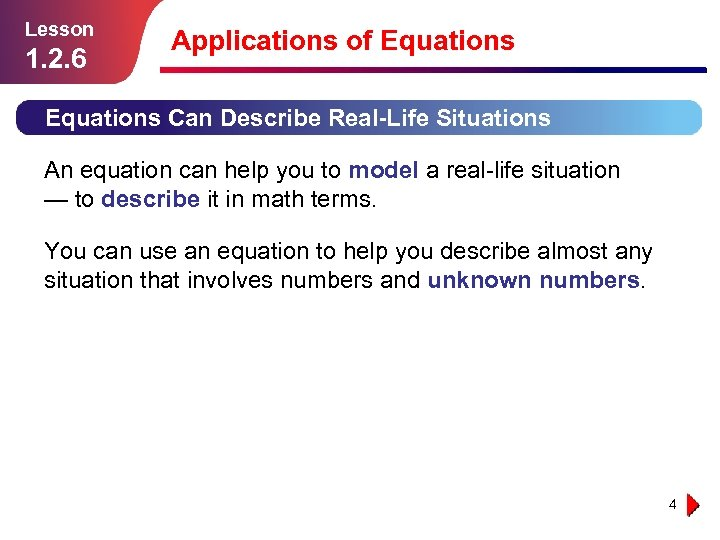Lesson 1. 2. 6 Applications of Equations Can Describe Real-Life Situations An equation can help you to model a real-life situation — to describe it in math terms. You can use an equation to help you describe almost any situation that involves numbers and unknown numbers. 4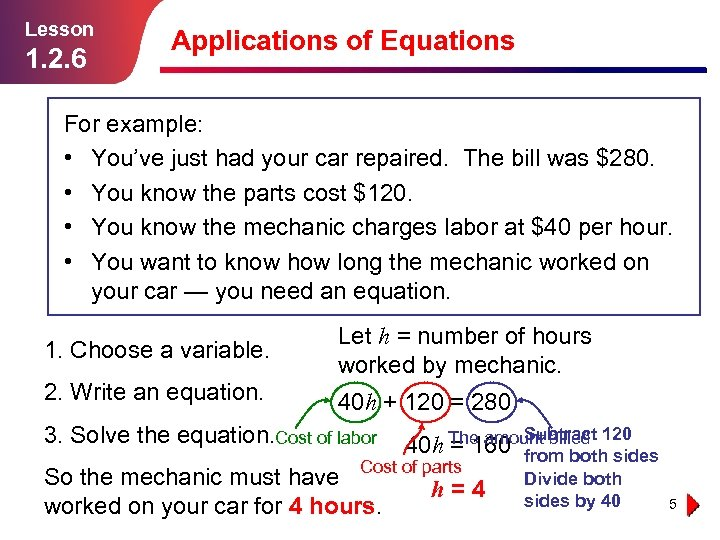Lesson 1. 2. 6 Applications of Equations For example: • You’ve just had your car repaired. The bill was \$280. • You know the parts cost \$120. • You know the mechanic charges labor at \$40 per hour. • You want to know how long the mechanic worked on your car — you need an equation. 1. Choose a variable. Let h = number of hours worked by mechanic. 2. Write an equation. 40 h + 120 = 280 3. Solve the equation. Cost of labor Subtract 120 The amount billed 40 h = 160 from both sides Cost of parts So the mechanic must have worked on your car for 4 hours. h = 4 Divide both sides by 40 5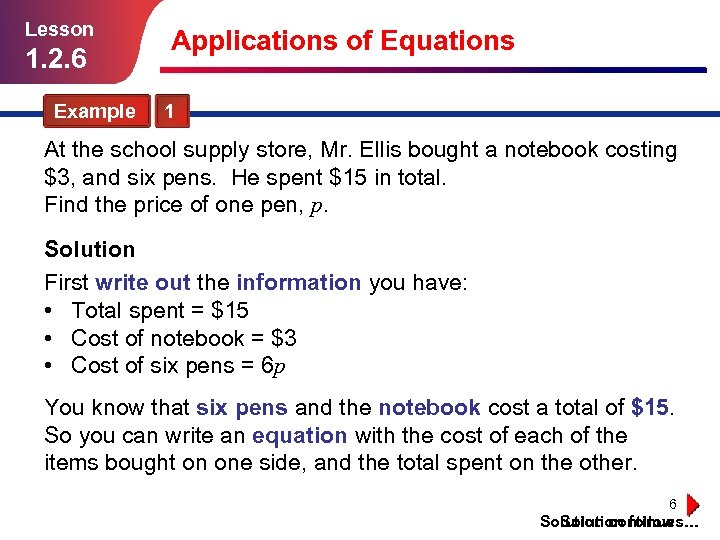Lesson 1. 2. 6 Applications of Equations Example 1 At the school supply store, Mr. Ellis bought a notebook costing \$3, and six pens. He spent \$15 in total. Find the price of one pen, p. Solution First write out the information you have: • Total spent = \$15 • Cost of notebook = \$3 • Cost of six pens = 6 p You know that six pens and the notebook cost a total of \$15. So you can write an equation with the cost of each of the items bought on one side, and the total spent on the other. 6 Solution continues… Solution follows…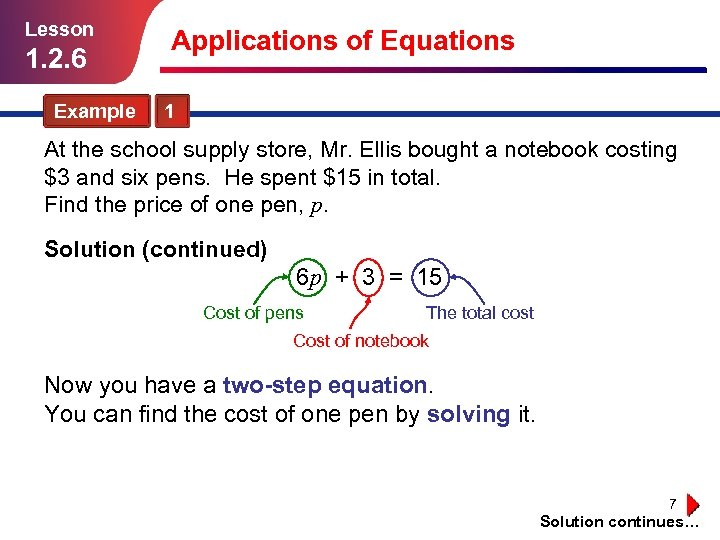Lesson 1. 2. 6 Applications of Equations Example 1 At the school supply store, Mr. Ellis bought a notebook costing \$3 and six pens. He spent \$15 in total. Find the price of one pen, p. Solution (continued) 6 p + 3 = 15 Cost of pens The total cost Cost of notebook Now you have a two-step equation. You can find the cost of one pen by solving it. 7 Solution continues…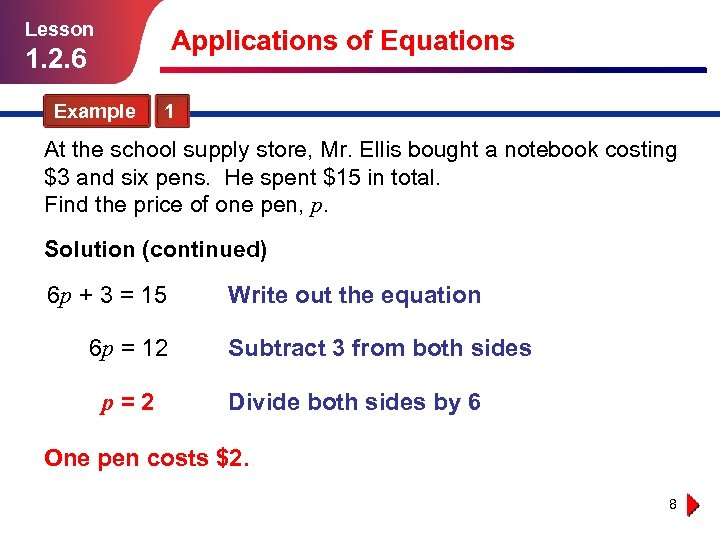Lesson Applications of Equations 1. 2. 6 Example 1 At the school supply store, Mr. Ellis bought a notebook costing \$3 and six pens. He spent \$15 in total. Find the price of one pen, p. Solution (continued) 6 p + 3 = 15 6 p = 12 p = 2 Write out the equation Subtract 3 from both sides Divide both sides by 6 One pen costs \$2. 8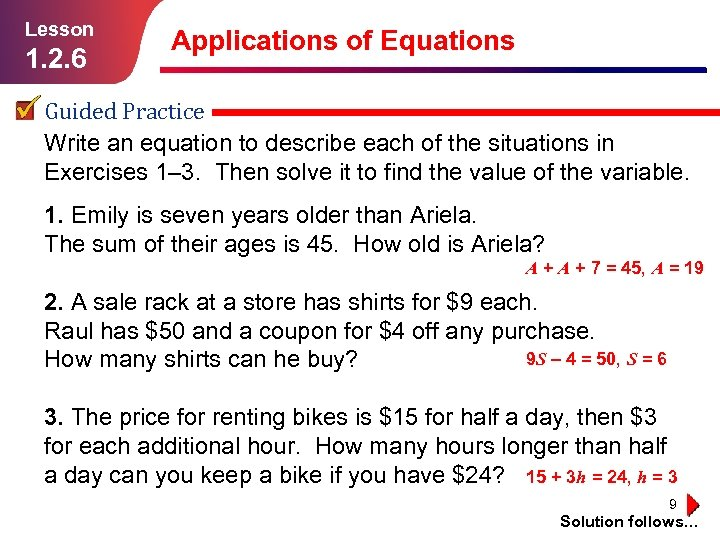Lesson 1. 2. 6 Applications of Equations Guided Practice Write an equation to describe each of the situations in Exercises 1– 3. Then solve it to find the value of the variable. 1. Emily is seven years older than Ariela. The sum of their ages is 45. How old is Ariela? A + 7 = 45, A = 19 2. A sale rack at a store has shirts for \$9 each. Raul has \$50 and a coupon for \$4 off any purchase. 9 S – 4 = 50, S = 6 How many shirts can he buy? 3. The price for renting bikes is \$15 for half a day, then \$3 for each additional hour. How many hours longer than half a day can you keep a bike if you have \$24? 15 + 3 h = 24, h = 3 9 Solution follows…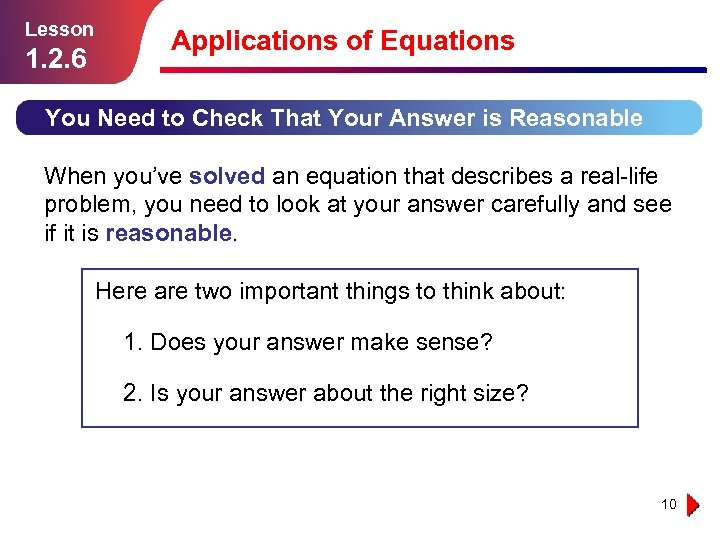Lesson 1. 2. 6 Applications of Equations You Need to Check That Your Answer is Reasonable When you’ve solved an equation that describes a real-life problem, you need to look at your answer carefully and see if it is reasonable. Here are two important things to think about: 1. Does your answer make sense? 2. Is your answer about the right size? 10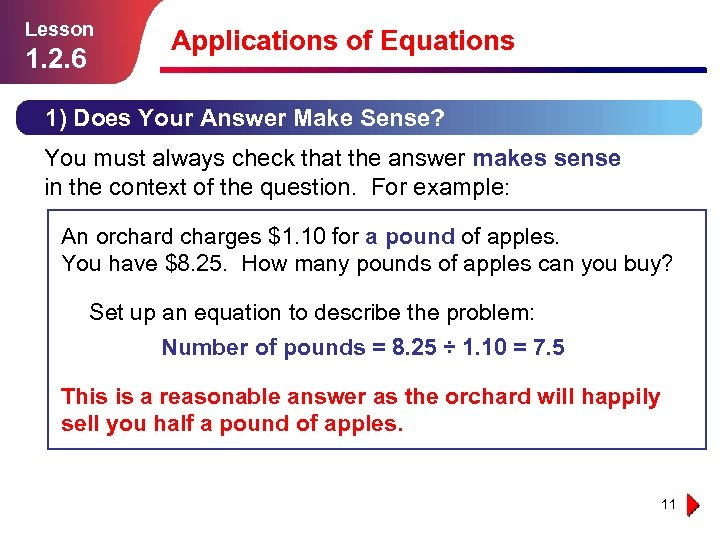Lesson 1. 2. 6 Applications of Equations 1) Does Your Answer Make Sense? You must always check that the answer makes sense in the context of the question. For example: An orchard charges \$1. 10 for a pound of apples. You have \$8. 25. How many pounds of apples can you buy? Set up an equation to describe the problem: Number of pounds = 8. 25 ÷ 1. 10 = 7. 5 This is a reasonable answer as the orchard will happily sell you half a pound of apples. 11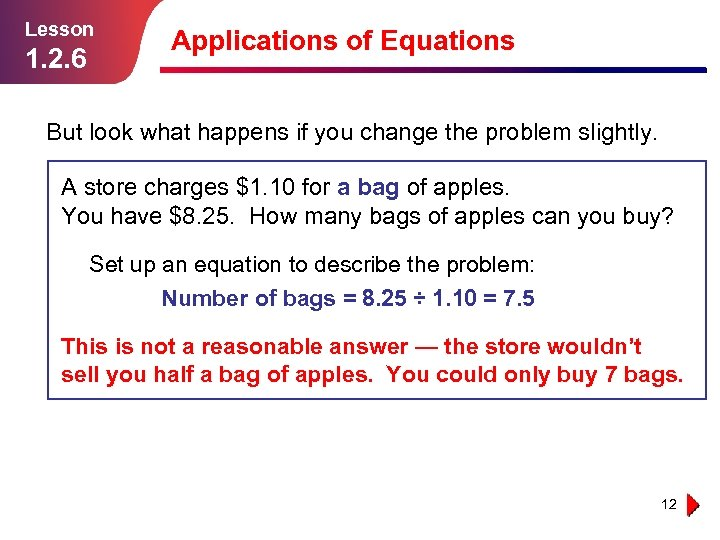Lesson 1. 2. 6 Applications of Equations But look what happens if you change the problem slightly. A store charges \$1. 10 for a bag of apples. You have \$8. 25. How many bags of apples can you buy? Set up an equation to describe the problem: Number of bags = 8. 25 ÷ 1. 10 = 7. 5 This is not a reasonable answer — the store wouldn’t sell you half a bag of apples. You could only buy 7 bags. 12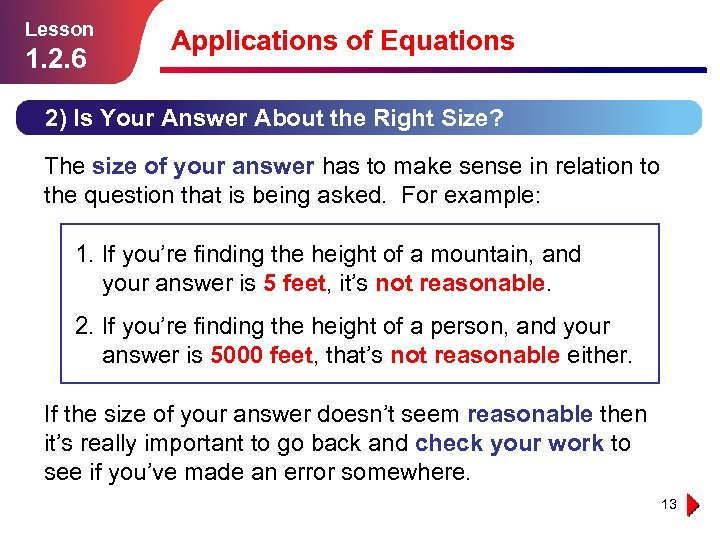Lesson 1. 2. 6 Applications of Equations 2) Is Your Answer About the Right Size? The size of your answer has to make sense in relation to the question that is being asked. For example: 1. If you’re finding the height of a mountain, and your answer is 5 feet, it’s not reasonable. 2. If you’re finding the height of a person, and your answer is 5000 feet, that’s not reasonable either. If the size of your answer doesn’t seem reasonable then it’s really important to go back and check your work to see if you’ve made an error somewhere. 13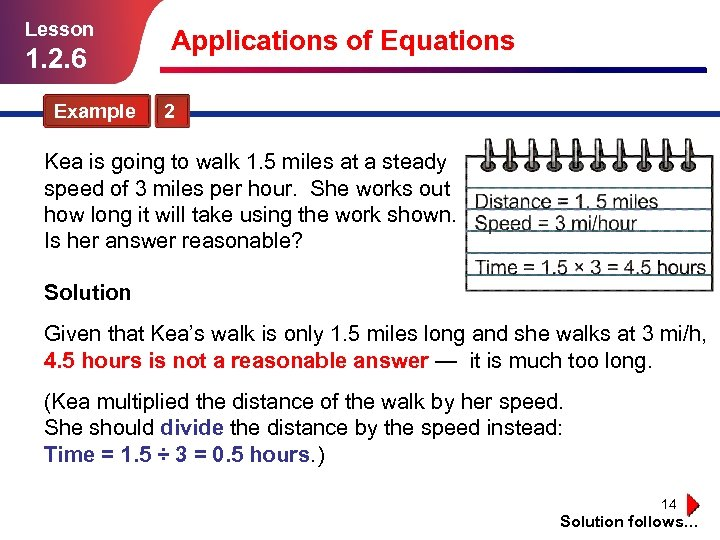Lesson 1. 2. 6 Applications of Equations Example 2 Kea is going to walk 1. 5 miles at a steady speed of 3 miles per hour. She works out how long it will take using the work shown. Is her answer reasonable? Solution Given that Kea’s walk is only 1. 5 miles long and she walks at 3 mi/h, 4. 5 hours is not a reasonable answer — it is much too long. (Kea multiplied the distance of the walk by her speed. She should divide the distance by the speed instead: Time = 1. 5 ÷ 3 = 0. 5 hours. ) 14 Solution follows…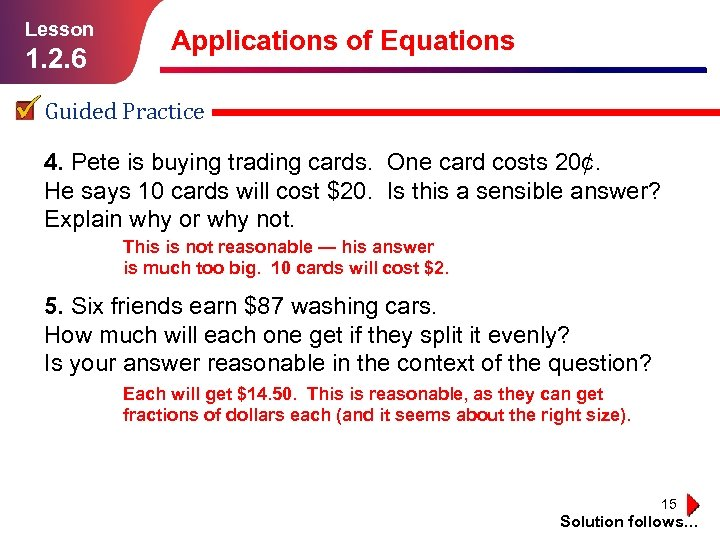Lesson 1. 2. 6 Applications of Equations Guided Practice 4. Pete is buying trading cards. One card costs 20¢. He says 10 cards will cost \$20. Is this a sensible answer? Explain why or why not. This is not reasonable — his answer is much too big. 10 cards will cost \$2. 5. Six friends earn \$87 washing cars. How much will each one get if they split it evenly? Is your answer reasonable in the context of the question? Each will get \$14. 50. This is reasonable, as they can get fractions of dollars each (and it seems about the right size). 15 Solution follows…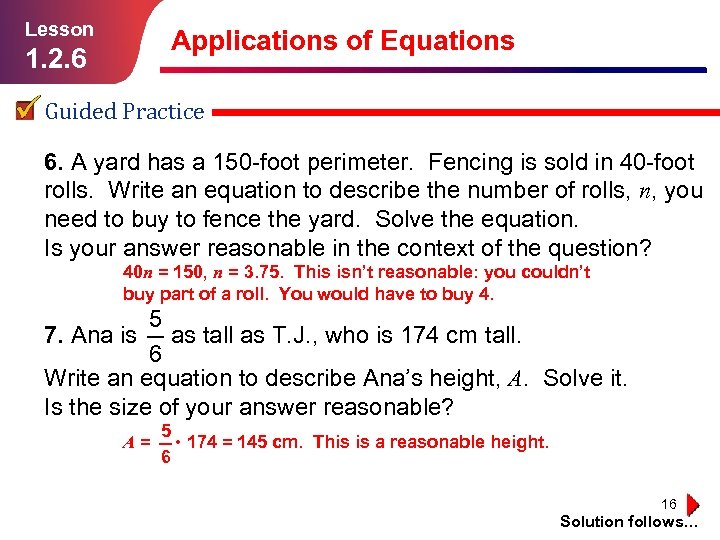Lesson 1. 2. 6 Applications of Equations Guided Practice 6. A yard has a 150 -foot perimeter. Fencing is sold in 40 -foot rolls. Write an equation to describe the number of rolls, n, you need to buy to fence the yard. Solve the equation. Is your answer reasonable in the context of the question? 40 n = 150, n = 3. 75. This isn’t reasonable: you couldn’t buy part of a roll. You would have to buy 4. 5 7. Ana is as tall as T. J. , who is 174 cm tall. 6 Write an equation to describe Ana’s height, A. Solve it. Is the size of your answer reasonable? 5 A = • 174 = 145 cm. This is a reasonable height. 6 16 Solution follows…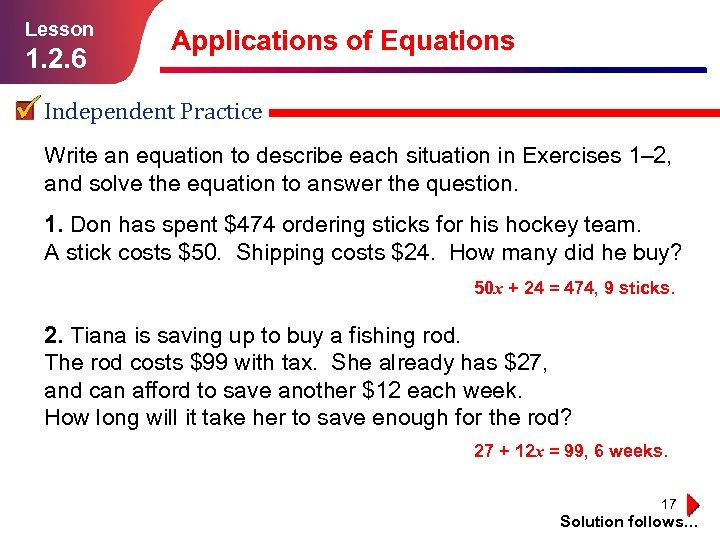Lesson 1. 2. 6 Applications of Equations Independent Practice Write an equation to describe each situation in Exercises 1– 2, and solve the equation to answer the question. 1. Don has spent \$474 ordering sticks for his hockey team. A stick costs \$50. Shipping costs \$24. How many did he buy? 50 x + 24 = 474, 9 sticks. 2. Tiana is saving up to buy a fishing rod. The rod costs \$99 with tax. She already has \$27, and can afford to save another \$12 each week. How long will it take her to save enough for the rod? 27 + 12 x = 99, 6 weeks. 17 Solution follows…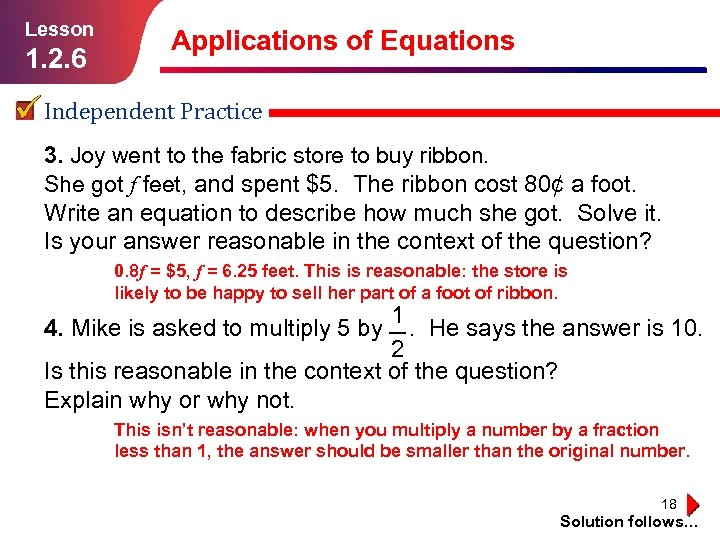Lesson 1. 2. 6 Applications of Equations Independent Practice 3. Joy went to the fabric store to buy ribbon. She got f feet, and spent \$5. The ribbon cost 80¢ a foot. Write an equation to describe how much she got. Solve it. Is your answer reasonable in the context of the question? 0. 8 f = \$5, f = 6. 25 feet. This is reasonable: the store is likely to be happy to sell her part of a foot of ribbon. 1 4. Mike is asked to multiply 5 by . He says the answer is 10. 2 Is this reasonable in the context of the question? Explain why or why not. This isn’t reasonable: when you multiply a number by a fraction less than 1, the answer should be smaller than the original number. 18 Solution follows…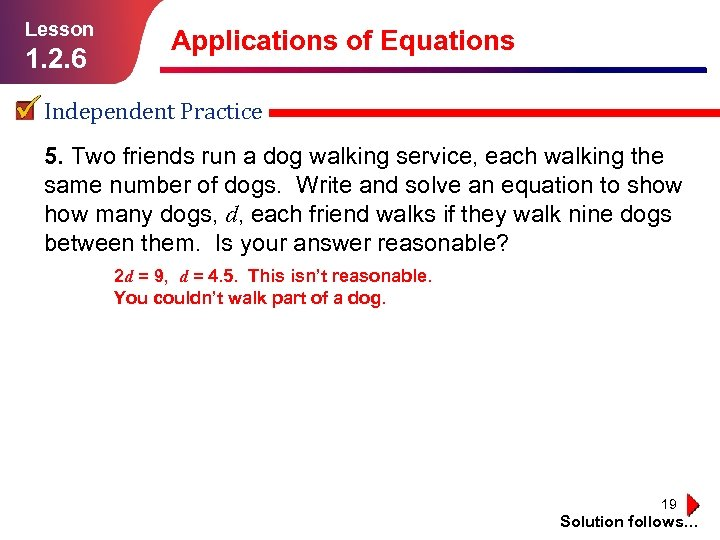Lesson 1. 2. 6 Applications of Equations Independent Practice 5. Two friends run a dog walking service, each walking the same number of dogs. Write and solve an equation to show many dogs, d, each friend walks if they walk nine dogs between them. Is your answer reasonable? 2 d = 9, d = 4. 5. This isn’t reasonable. You couldn’t walk part of a dog. 19 Solution follows…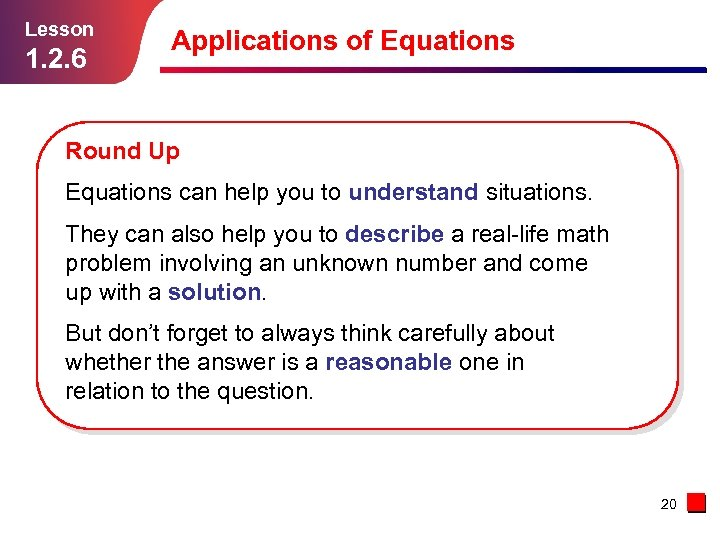Lesson 1. 2. 6 Applications of Equations Round Up Equations can help you to understand situations. They can also help you to describe a real-life math problem involving an unknown number and come up with a solution. But don’t forget to always think carefully about whether the answer is a reasonable one in relation to the question. 20